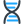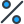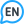• # Iron has a density of 7.86 g/cm3 . Calculate the volume (in dL ) of a piece of iron having a mass of 4.89 kg . Note that the density is provided in different units of volume and mass than the desired units of volume ( dL ) and the given units of mass ( kg ). You will need to express the density in kg/dL ( 1 cm3 = 1 mL ) before calculating the volume for the piece of iron.

•Biology
5 minutes ago
You can only transport polar substances using
•Mathematics
5 minutes ago
Use the gcf and the distributive property to find the sum of 15+36
•English
5 minutes ago
The temperature was almost 100° yesterday and I was working outside of four hours by the end of the day I was so hot my eyes motor out of my face
•Mathematics
5 minutes ago
21 + 39 = ? (7 + 13) someone pls help me understand this!
•Mathematics
5 minutes ago
Solve the following proportion: 6/8=8/a
Information

Visitors in the Guests group cannot leave comments on this post.